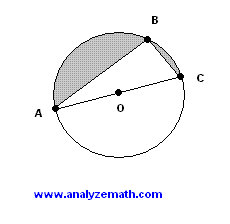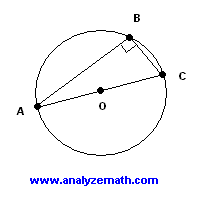# Triangle Inscribed in a Circle - Problem With Solution

Inscribed right triangle problem with detailed solution.

## Problem

In the figure below, triangle ABC is a triangle inscribed inside the circle of center O and radius r = 10 cm. Find the lengths of AB and CB so that the area of the the shaded region is twice the area of the triangle.Solution to Problem :

• If the center O is on AC then AC is a diameter of the circle and the triangle has a right angle at B (Thales's theorem). If At is the area of triangle ABC and As the shaded area then we need to have As = 2 At.• We also have.
At + As = (1/2) area of circle = (1/2) Pi 10 2 = 50 Pi
At + 2 At = 50 Pi
• Which gives.
At = 50 Pi / 3
• Since triangle ABC has a right angle, we now use the internal angle (to the triangle) A to write.
sin(A) = CB / AC = CB / 20 which gives CB = 20 sin (A)
and cos(A) = AB / AC = AB / 20 which gives AB = 20 cos (A)
• The area At might also be written as follows (using the identity sin(2A) = 2 sin (A) cos (A)).
At = (1/2) * AB * CB = 200 cos (A) sin(A) = 100 sin (2A) = 50 Pi / 3
• The above equation gives.
sin (2A) = 0.5 Pi / 3
• Use calculator to solve for 2A. Two solutions
2A = 31.6 degrees (nearest tenth) or A = 15.8 degrees
2A = 148.4 degrees (nearest tenth) or A = 74.2 degrees
• We now calculate the lengths of AB and CB. Two solutions
first solution
AB = 20 cos (15.8) = 19.24 (2 decimals) and CB = 20 sin (15.8) = 5.45 (2 decimals)
second solution
AB = 20 cos (74.2) = 5.45 (2 decimals) and CB = 20 sin (74.2) = 19.24 (2 decimals)
• The two solutions correspond to two congruent right triangles.

## More References and Links to Geometry

Geometry Tutorials, Problems and Interactive Applets.